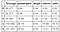# IntroductionFigure 1. Illustrating the Machine Learning Process. Image by Benjamin O. Tayo.

In this article, we present a practical tutorial of the machine learning process using the cruise ship dataset cruise_ship_info.csv. The dataset and Jupyter notebook for this tutorial can be downloaded from here: https://github.com/bot13956/Machine_Learning_Process_Tutorial.

# 1. Problem Framing

Objective: The goal of this project is to build a regressor model that recommends the “crew” size for potential cruise ship buyers using the cruise ship dataset cruise_ship_info.csv.

# 2. Data Analysis

## 2.1 Import necessary libraries

`import numpy as np import pandas as pd import matplotlib.pyplot as plt import seaborn as sns`

## 2.2 Read dataset and display columns

`df = pd.read_csv("cruise_ship_info.csv")df.head()`

## 2.3 Calculate the covariance matrix

`cols = ['Age', 'Tonnage', 'passengers', 'length',                       'cabins','passenger_density','crew']from sklearn.preprocessing import StandardScalerstdsc = StandardScaler()X_std = stdsc.fit_transform(df[cols].iloc[:,range(0,7)].valuescov_mat = np.cov(X_std.T)`

## 2.4 Generate a heatmap for visualizing the covariance matrix

`plt.figure(figsize=(10,10))sns.set(font_scale=1.5)hm = sns.heatmap(cov_mat,                 cbar=True,                 annot=True,                 square=True,                 fmt='.2f',                 annot_kws={'size': 12},                 yticklabels=cols,                 xticklabels=cols)plt.title('Covariance matrix showing correlation coefficients')plt.tight_layout()plt.show()`

## 2.5 Feature selection using covariance matrix plot

`cols_selected = ['Tonnage', 'passengers', 'length', 'cabins','crew']df[cols_selected].head()`Table 2. First 5 rows of important features and predictor variable.

## 2.6 Define your features matrix and target variable

`X = df[cols_selected].iloc[:,0:4].values    # features matrix  y = df[cols_selected]['crew'].values        # target variable`

The features matrix and the target variable obtained above can then be used for model building.

# 3. Model Building

Since our goal is to use regression, we will implement 3 different regression algorithms: Linear Regression (LR), KNeighbors Regression (KNR), and Support Vector Regression (SVR).

The data set has to be divided into training, validation, and test sets. Hyperparameter tuning is used to fine-tune the model in order to prevent overfitting. Cross-validation is performed to ensure the model performs well on the validation set. After fine-tuning model parameters, the model is applied to the test data set. The model’s performance on the test data set is approximately equal to what would be expected when the model is used for making predictions on unseen data.

## 3.1 Model building and evaluation

`from sklearn.metrics import r2_scorefrom sklearn.model_selection import cross_val_scorefrom sklearn.model_selection import train_test_splitfrom sklearn.preprocessing import StandardScalerfrom sklearn.linear_model import LinearRegressionfrom sklearn.neighbors import KNeighborsRegressorfrom sklearn.svm import SVRfrom sklearn.pipeline import Pipelinepipe_lr = Pipeline([('scl', StandardScaler()),('lr',                         LinearRegression())])pipe_knr = Pipeline([('scl', StandardScaler()),('knr',                      KNeighborsRegressor(n_neighbors = 3))])pipe_svr = Pipeline([('scl', StandardScaler()),('svr',                      SVR(kernel='linear',C=1.0))])sc_y = StandardScaler()train_score_lr = []train_score_knr =  []train_score_svr =  []n = 15for i in range(n):    X_train, X_test, y_train, y_test = train_test_split( X, y,                                       test_size=0.3, random_state=i)    y_train_std = sc_y.fit_transform(y_train[:,                                             np.newaxis]).flatten()    train_score_lr = np.append(train_score_lr,                                np.mean(cross_val_score(pipe_lr,                                X_train, y_train_std,                                scoring ='r2' , cv = 10)))    train_score_knr = np.append(train_score_knr,                                np.mean(cross_val_score(pipe_knr,                                X_train, y_train_std,                                scoring ='r2' , cv = 10)))    train_score_svr = np.append(train_score_svr,                                np.mean(cross_val_score(pipe_svr,                                X_train, y_train_std,                                 scoring ='r2' , cv = 10)))train_mean_lr = np.mean(train_score_lr)train_std_lr = np.std(train_score_lr)train_mean_knr = np.mean(train_score_knr)train_std_knr = np.std(train_score_knr)train_mean_svr = np.mean(train_score_svr)train_std_svr = np.std(train_score_svr)`

## 3.2 Output from machine learning model

`print('R2 train for lr: %.3f +/- %.3f' %                     (train_mean_lr,train_std_lr))print('R2 train for knn_lr: %.3f +/- %.3f' %                        (train_mean_knr,train_std_knr))print('R2 train for svm_lr: %.3f +/- %.3f' %                     (train_mean_svr,train_std_svr))`

## 3.3 Generate visualization of cross-validation score

`plt.figure(figsize=(15,11))plt.plot(range(n),train_score_lr,color='blue', linestyle='dashed',          marker='o',markerfacecolor='blue', markersize=10, label= 'Linear Regression')plt.plot(range(n),train_score_knr,color='green', linestyle='dashed',          marker='s',markerfacecolor='green', markersize=10, label = 'KNeighbors Regression')plt.plot(range(n),train_score_svr,color='red', linestyle='dashed',          marker='^',markerfacecolor='red', markersize=10, label = 'Support Vector Regression')plt.grid()plt.ylim(0.7,1)plt.title ('Mean cross-validation R2 score vs. random state parameter', size = 14)plt.xlabel('Random state parameter', size = 14)plt.ylabel('Mean cross-validation R2 score', size = 14)plt.legend()plt.show()`

## 3.4 Model’s performance on test set

`pipe_lr.fit(X_train, y_train_std)pipe_knr.fit(X_train, y_train_std)pipe_svr.fit(X_train, y_train_std)r2_score_lr = r2_score(y_test,                    sc_y.inverse_transform(pipe_lr.predict(X_test)))r2_score_knr = r2_score(y_test,                    sc_y.inverse_transform(pipe_knr.predict(X_test)))r2_score_svr = r2_score(y_test,                   sc_y.inverse_transform(pipe_svr.predict(X_test)))print('R2 test for lr:  %.3f ' % r2_score_lr)print('R2 test for knr: %.3f ' % r2_score_knr)print('R2 test for svr: %.3f ' % r2_score_svr)`

# 4. Application

In this stage, the final machine learning model is selected and put into production. The model is evaluated in a production setting in order to assess its performance. Any mistakes encountered when transforming from an experimental model to its actual performance on the production line has to be analyzed. This can then be used in fine-tuning the original model.

Based on the results from section 3, we observe that Linear Regression and Support Vector Regression perform almost at same level, and better than KNeighbors Regression. So the final model selected could either be Linear Regression or Support Vector Regression.

In summary, we have discussed the main stages of a machine learning process. This tutorial illustrates the practical steps involved in the machine learning process. We have illustrated our calculations using a regression problem, but the same process is applicable for classification projects equally.

# Additional Data Science/Machine Learning Resources

Data Science Curriculum

Essential Maths Skills for Machine Learning

3 Best Data Science MOOC Specializations

5 Best Degrees for Getting into Data Science

5 reasons why you should begin your data science journey in 2020

Theoretical Foundations of Data Science — Should I Care or Simply Focus on Hands-on Skills?

Machine Learning Project Planning

How to Organize Your Data Science Project

Productivity Tools for Large-scale Data Science Projects

A Data Science Portfolio is More Valuable than a Resume

Data Science 101 — A Short Course on Medium Platform with R and Python Code Included

For questions and inquiries, please email me: benjaminobi@gmail.com

Written by

Written by

## Benjamin Obi Tayo Ph.D.

#### Physicist, Data Science Educator, Writer. Interests: Data Science, Machine Learning, AI, Python & R, Predictive Analytics, Materials Sciences, Biophysics